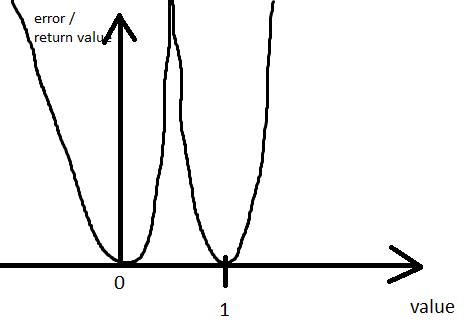# Looking for loss function to enforce binary (near 0 or 1) output

Hi there,
I’m looking for a loss function which will motivate a network to output on the last layer either near 0 or near 1 values. I’m imagining a function that looks similar to this. The output layer is of type float/double and will be used as a mask.Background:
I’m training an unsupervised network to predict masks. My evaluation function would ideally get near 0 or 1 values. It can handle anything, but it would make the most sense if it was 0 or 1. I’m planning to sum the loss function, which will motivate 0/1 values, with the loss of my evaluation function. While clamping to [0, 1] would solve the left and right side, it would not help with values in between.

I cannot use the Binary Cross Entropy loss because I do not have the target values.

While I could use a combination of torch.gt and clamping I don’t know if this would work as well as it would allow the model to be “uncertain” i.e. output values which are extremely close to 0.5 e.g. 0.499999999 or 0.50000000001. Please tell me if my reasoning here is flawed.

If anyone has any better ideas on how to solve the 0/1 mask problem other than with such a loss function, I’m all open ears!

Cheers!

Hi Metro!

I don’t understand your use case, but, in general, you can certainly
penalize outputs that are not close to 0 or 1.

Let `x` be a single output. You could then try things like:

``````penalty_0_1 = (x * (1.0 - x))**2
penalty_0_1 = torch.abs (x * (1.0 - x))
penalty_0_1 = ((x * (1.0 - x))**2)**alpha
``````

where the last version includes the parameter `alpha` that can be
used to tune the shape of the “wells” at 0 and 1.

(`x` would likely be a pytorch tensor, so you would typically sum
after calculating the penalty to get a single scalar penalty that would
push the individual elements of the tensor towards 0 or 1.)

There is no (reasonable) way to avoid having an “uncertain” point
somewhere between 0 and 1 (most likely at 0.5). But more importantly,
you don’t want the penalty at 0.5 to be so large that it is impractical
for the network output to move from near 0 to near 1 or vice versa.

Suppose that the network output “should” be (near) 1, but happens
to start out near 0. As the network trains, you want it to be able to
get over the penalty hump, and not stay stuck near 0 forever. (One
approach to this issue is to multiply your penalty with a weight. At
the beginning of training start with a small weight so that the network
output can move easily between 0 and 1, but increase the weight as
training progresses so that the network output is forced increasingly
close to 0 or 1.)

Best.

K. Frank

1 Like